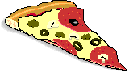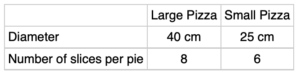# Dividing PizzaLast night I ate 3 slices of pizza for dinner. Is that a lot? You would need more information about the size of the pizza and the number of slices in the whole pie before you could decide.

My local pizzeria has two sizes of pizzas, small and large. Use the information from the chart below to decide how many slices of the small pizza would be equal to or greater than 3 slices from the large pizza. (You may assume that the two sizes are of equal thickness.)Extension
How would you compare the cost of the two pizzas?